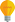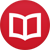# How to define functions without using variables

by Jakub MarianTip: See my list of theMost Common Mistakes in English. It will teach you how to avoid mis­takes with com­mas, pre­pos­i­tions, ir­reg­u­lar verbs, and much more.

First, note that this article is not about the definition of elementary functions, such as $\sin$ and $\cos$, and operations, like $a^b$, but rather about the way to define a new function using elementary functions, e.g. $f(x) = x^2e^x$, without the need to use variables.

There already is a way to denote functions without variables for many of them; for example, if $A$ is an operator which can be applied to a real function, you can write just $A(\sin)$ instead of $A(f)$, $f(x) = \sin(x)$. The reason why we generally don’t do that, i.e. why you are very unlikely to meet “$\sin$” without an argument (at least in the mathematical world) is that it would lead to an inconsistency in notation. For example, if $f(x) = \sin(x^2)$, how would you denote $A(f)$ without defining $f$ first using variables?

The solution is actually very simpledefine a new function:

$$\iota(x) = x\,.$$

Here I intentionally don’t write to which set the $x$ belongs. It can be $\iota:ℝ→ℝ$, $\iota:ℂ→ℂ$ or any other you find appropriate. I’ve chosen the symbol $\iota$ (the greek letter iota) because it is the identity function, i.e. the function that “does nothing”, and it is already common to denote the inclusion from a set to its superset (i.e. the function that “does nothing”) by iota.

Using $\iota$, we can denote any function without using variables. We will follow two rules:

1. If $*$ is a binary operation and $f$ and $g$ are functions, then $f*g$ is a function defined by $$(f*g)(x) = f(x)*g(x)\,.$$ In particular, $fg$ means $(fg)(x) = f(x)g(x)$ and $\l(÷{f}{g}\r)(x) = ÷{f(x)}{g(x)}$. Also, for the operation of exponentiation, we let $f^g$ denote the function $$(f^g)(x) = (f(x))^{g(x)}\,.$$
2. If $f$ and $g$ are functions, then the composition of $f$ and $g$, i.e. $f ∘ g$, may be denoted $f[g]$.

For example, using the first rule:

$$\text{If}\quad\begin{array}[l] \phantom{} f = \iota^3 \\ g = \sin^2 \\ h = \sin\cos \\ i = e^\iota \\ j = ÷{\sin}{\cos} \end{array}\quad\text{then}\quad\begin{array}[l] \phantom{} f(x) = x^3 \\ g(x) = (\sin(x))^2 = \sin^2(x) \\ h(x) = \sin(x)\cos(x) \\ i(x) = e^x \\ j(x) = ÷{\sin(x)}{\cos(x)} \end{array}$$

And using the second rule:

$$\text{If}\quad\begin{array}[l] \phantom{} f = \sin[\cos] \\ g = \tan[\iota^2+1] \\ h = \log\l[÷{\sin}{\cos}\r]\end{array}\quad\text{then}\quad\begin{array}[l] \phantom{} f(x) = \sin(\cos(x)) \\ g(x) = \tan(x^2+1) \\ h(x) = \log\l(\l(÷{\sin}{\cos}\r)(x)\r) = \log\l(÷{\sin(x)}{\cos(x)}\r)\end{array}$$

Of course, the second rule is optional because we can always write $f ∘ g$ instead of $f[g],$ but using brackets is often more naturalwhich of the following expressions do you consider more legible?

$$\sin[\iota^2]\cos[\iota^3] = ({\sin} ∘ \iota^2)({\cos} ∘ \iota^3)$$

Using square brackets instead of round parentheses allows us to distinguish between multiplication and composition. Compare

$$(\cos+\sin)(\cos-\sin) = \cos^2-\sin^2$$

with

$$({\cos}+{\sin})[{\cos}-{\sin}] = \cos[{\cos}-{\sin}] + \sin[{\cos}-{\sin}]\,,$$

## What is this all good for?

Maybe you are wondering why we should even bother to define functions without using variables. Consider the following function:

$$f = (\iota^2+\iota^3+\iota^4)∘(2\iota^2+3\iota^3+4\iota^4)∘(\iota+\iota^2)$$

Its structure is clear. However, in the standard notation, there’s no way to write $f$ using a simple formula. Subsequent polynomials are simply put “inside” the preceding ones:

\begin{align*} f(x) = &\big(2(x+x^2)^2+3(x+x^2)^3+4(x+x^2)^4\big)^2+\\ &+\big(2(x+x^2)^2+3(x+x^2)^3+4(x+x^2)^4\big)^3+\\ &+\big(2(x+x^2)^2+3(x+x^2)^3+4(x+x^2)^4\big)^4\,, \end{align*}

which is just a mess. In the former notation, it wouldn’t be hard to compute the value of $f$ at some point using only a pen and paper, for example

$$f(1) =(\iota^2+\iota^3+\iota^4) \l((2\iota^2+3\iota^3+4\iota^4)(2)\r) = (\iota^2+\iota^3+\iota^4)(96) \approx 86\cdot10^6 \ ,$$

but it would be a dull task in the standard notation. And not only is the task dull, it is also much more demanding on computational resources when performed using a computer.

The simplification does not lie only in computation of numerical values, but also in symbolic manipulations. For example, using the chain rule for derivatives

$$(f∘g∘h)’ = (f’∘g∘h)(g’∘h)h’$$

we can compute

\begin{align*} f’ = &\l((2\iota+3\iota^2+4\iota^3)∘(2\iota^2+3\iota^3+4\iota^4)∘(\iota+\iota^2)\r)\\ &\l((4\iota+9\iota^2+16\iota^3)∘(\iota+\iota^2)\r)(1+2\iota)\,. \end{align*}

Can you imagine calculating the derivative in the traditional notation?

By the way, I have written several educational ebooks. If you get a copy, you can learn new things and support this website at the same time—why don’t you check them out?